Moving regions of code which will do some level of mod math on overlapping sections.

### Algorithm

• Spawn a few shapes and assign them color indexes and velocities
• With each iteration:
• Find all of the overlapping intersections and sum the color indexes in those regions
• Take the resulting color index and wrap it around by the number of colors in the palette ( index % NUM_COLORS)
• Calculate where all of the shapes will be in the next step
• Draw it!

#### The Wrapped Color Palette Math

Consider the following color palette with the following assigned indexes:

When 2 colors are overlapped we ADD together the colliding colors and mod by the number of colors in the palette. This essentially creates a wrapped palette. For example:
• (0 + 1) % 5 = 1
• (4 + 1) % 5 = 0
• (3 + 4) % 5 = 2
• (3 + 4 + 1) % 5 = 3

This creates a sort of interesting result, since the relationships are not determined by any sort of color theory, but instead is based by the relationship of the indexes. it is also interesting, since some combinations may result in no change. (0 + 0 = 0). Here is am example as a simple overlap:

### Progression

#### Trying to get the overlaps right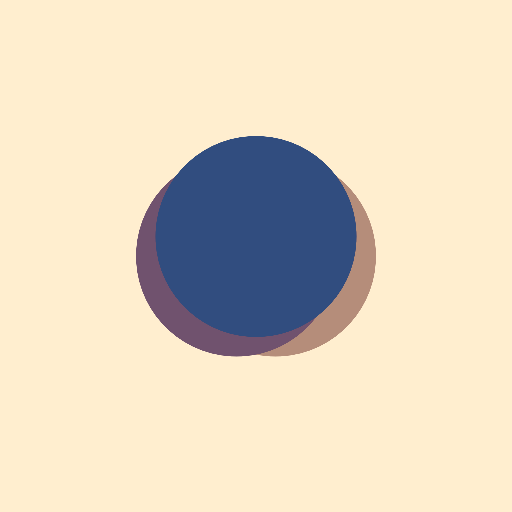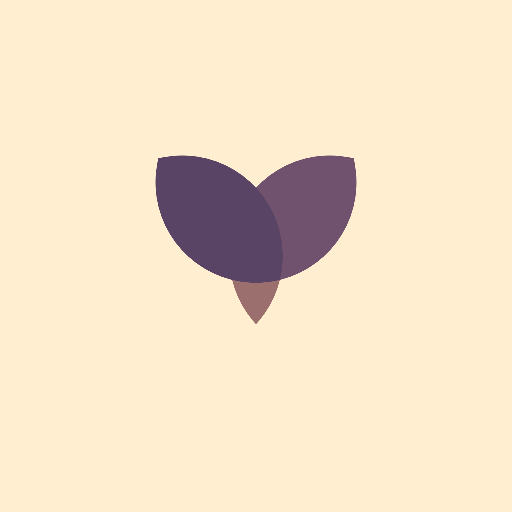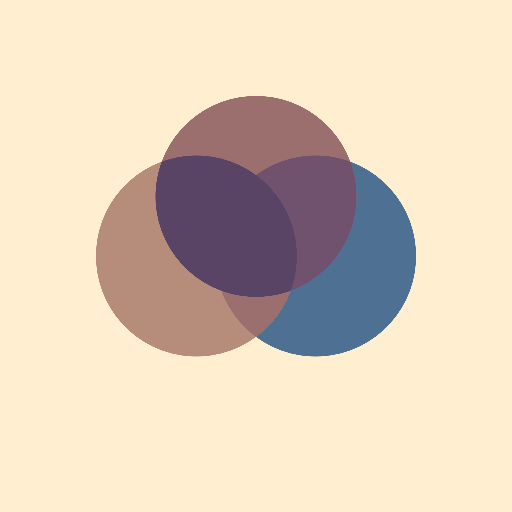#### All of the intersections calculated

I had to calculate all unique combinations of all of the shapes then construct the intersections and sort so that the intersection with the most shapes was on bottom (drawn last) so that it is not covered up by the other shapes.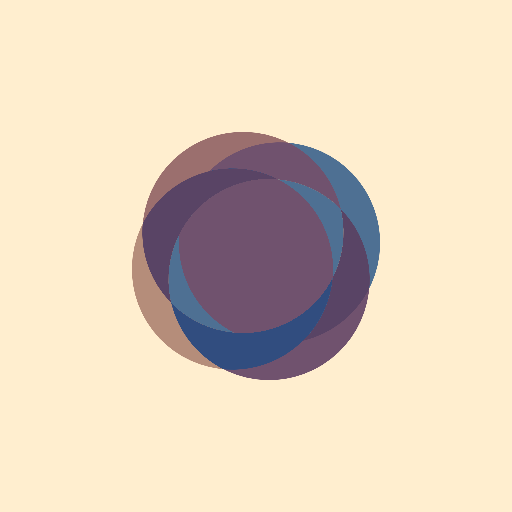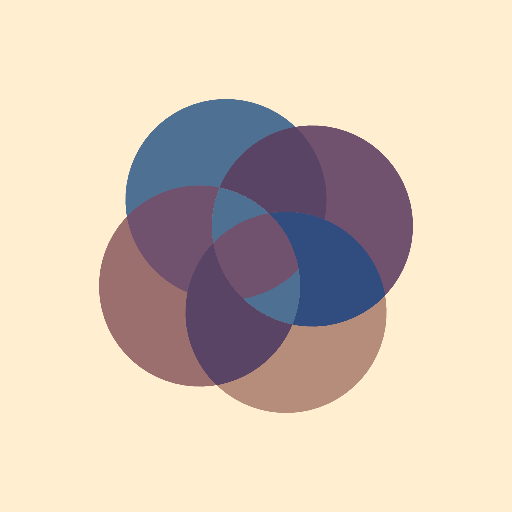#### Some randomness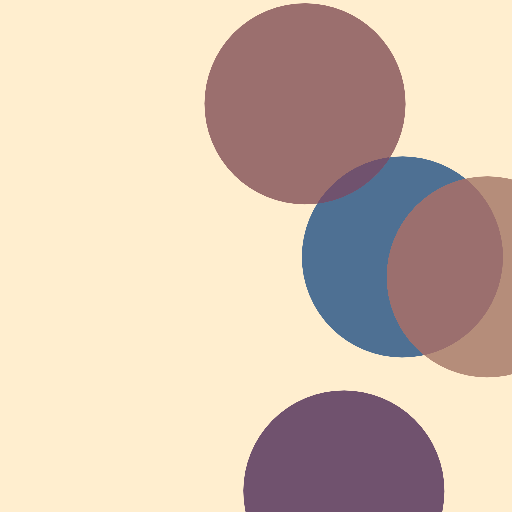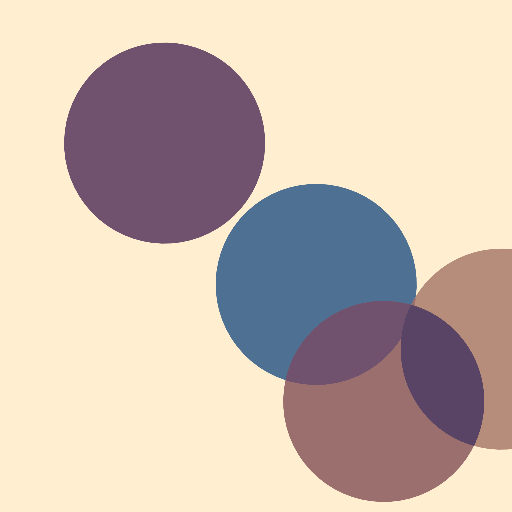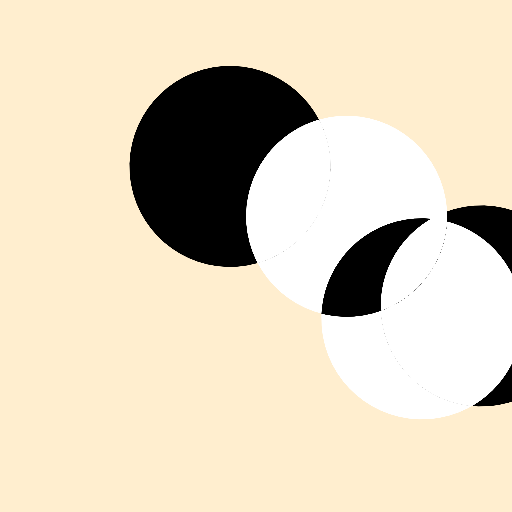One thing I noticed while playing with the black and white is any index of 0 will just nullify itself. So for example: (0 + 0) % 1 = 0, (1 + 1) % 2 = 0. I decided this logic is ok, but there is room for other logics here, or even just use of opacity, Shaders or some N-value logic or gradients.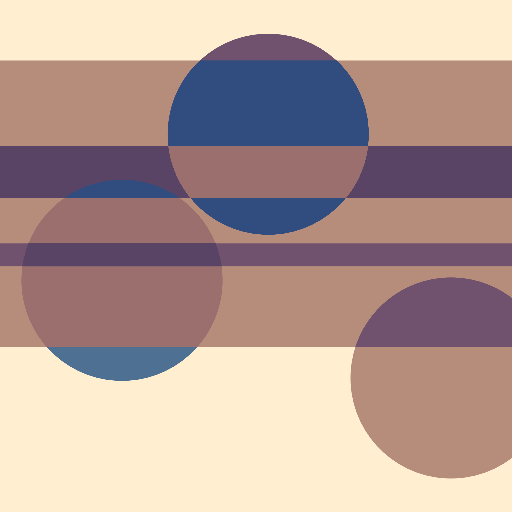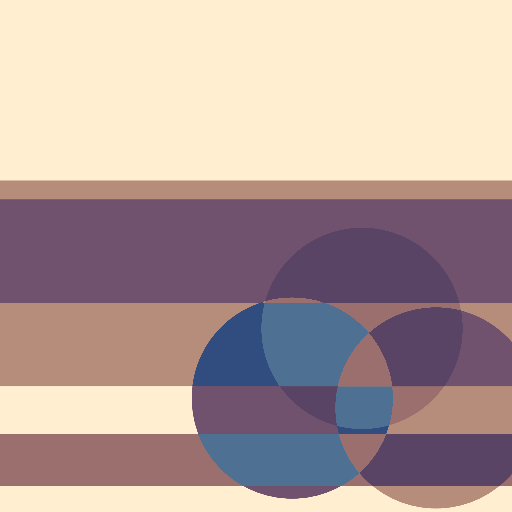#### Outlines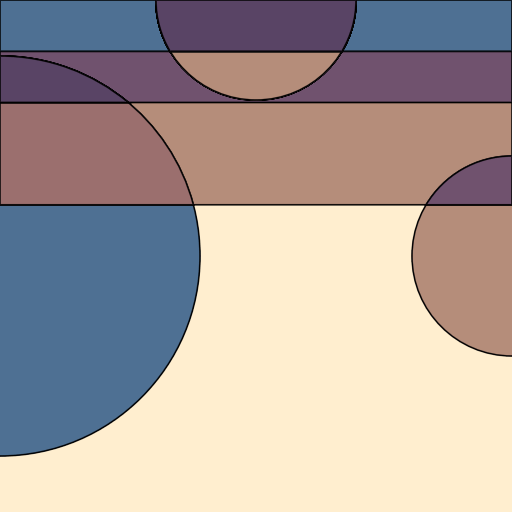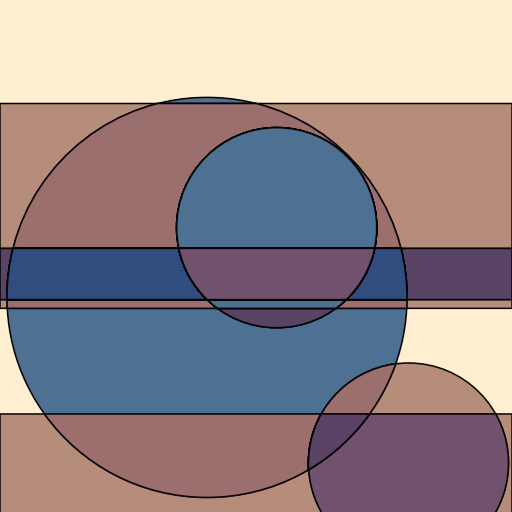#### Outline and Drawing Modes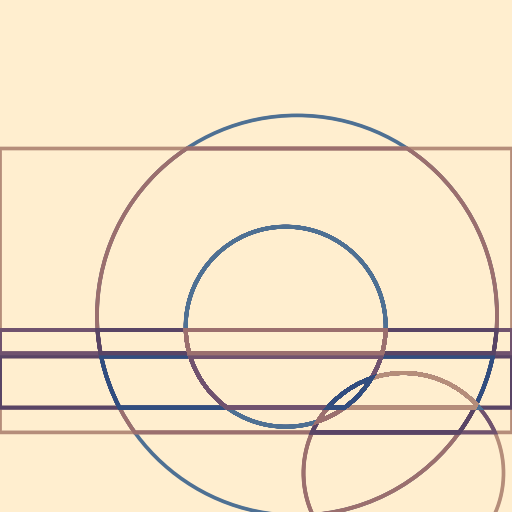### Future work

• Shaders to speed up th drawing
• play with opacity
• incorporate the background color into the shapes
• Random shapes instead of predestined shapes
• Gradients of mono-hue and saturation with variable value/brightness!? or even fixed s and v and mono hue Or hey we can just navigate through the color space and find the median distance between points.
• Sketch outlines coloring instead of the fills

Using OpenRNDR